Test: Electrical & Electronic Measurements- 7

# Test: Electrical & Electronic Measurements- 7 - Electrical Engineering (EE)

Test Description

## 10 Questions MCQ Test GATE Electrical Engineering (EE) 2023 Mock Test Series - Test: Electrical & Electronic Measurements- 7

Test: Electrical & Electronic Measurements- 7 for Electrical Engineering (EE) 2023 is part of GATE Electrical Engineering (EE) 2023 Mock Test Series preparation. The Test: Electrical & Electronic Measurements- 7 questions and answers have been prepared according to the Electrical Engineering (EE) exam syllabus.The Test: Electrical & Electronic Measurements- 7 MCQs are made for Electrical Engineering (EE) 2023 Exam. Find important definitions, questions, notes, meanings, examples, exercises, MCQs and online tests for Test: Electrical & Electronic Measurements- 7 below.
Solutions of Test: Electrical & Electronic Measurements- 7 questions in English are available as part of our GATE Electrical Engineering (EE) 2023 Mock Test Series for Electrical Engineering (EE) & Test: Electrical & Electronic Measurements- 7 solutions in Hindi for GATE Electrical Engineering (EE) 2023 Mock Test Series course. Download more important topics, notes, lectures and mock test series for Electrical Engineering (EE) Exam by signing up for free. Attempt Test: Electrical & Electronic Measurements- 7 | 10 questions in 30 minutes | Mock test for Electrical Engineering (EE) preparation | Free important questions MCQ to study GATE Electrical Engineering (EE) 2023 Mock Test Series for Electrical Engineering (EE) Exam | Download free PDF with solutions
 1 Crore+ students have signed up on EduRev. Have you?
Test: Electrical & Electronic Measurements- 7 - Question 1

### A voltage signal V(t) = A + B sin ωt is fed to an oscilloscope with the coupling mode set to DC, other settings being appropriate. The displayed wave can be expressed as

Detailed Solution for Test: Electrical & Electronic Measurements- 7 - Question 1

When a voltage signal is fed to an oscilloscope with DC coupling entire waveform will be displayed including the DC component.

Test: Electrical & Electronic Measurements- 7 - Question 2

### Calculate the ratio of vertical to horizontal frequencies for an oscilloscope which display the following Lissajous figure.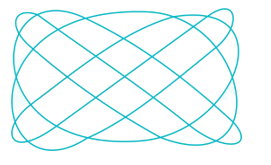Detailed Solution for Test: Electrical & Electronic Measurements- 7 - Question 2

Ratio of the two frequencies is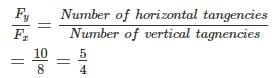*Answer can only contain numeric values
Test: Electrical & Electronic Measurements- 7 - Question 3

### A sinusoidal waveform, when observed on an oscilloscope, has a peak to peak amplitude of 4 cm. If the vertical sensitivity setting is 7.5 V/cm, the rms value of the voltage is__ (in V)

Detailed Solution for Test: Electrical & Electronic Measurements- 7 - Question 3

Vertical voltage sensitivity = 7.5 V/cm
Peak to peak voltage = 7.5 × 4 = 30 V
Maximum voltage = 15 V
Rms value of the voltage = 15/√2 = 10.6 V

Test: Electrical & Electronic Measurements- 7 - Question 4

A single channel analog oscilloscope is operated in X-Y mode. An ellipse rotating in anticlockwise direction and having major axis in 2nd and 4thquadrants displayed on the screen. The peak Y deflection is at 5 divisions and Y intercept is at 3 divisions. The phase difference between both the input signals is ______

Detailed Solution for Test: Electrical & Electronic Measurements- 7 - Question 4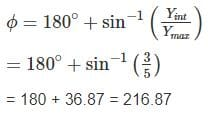Test: Electrical & Electronic Measurements- 7 - Question 5

An electrostatically deflected CRT has plane parallel deflecting plates which are 2.5 cm long and 0.5 cm apart. The distance of the screen form the center of the plates is 20 cm. The accelerating voltage is 2500 V. The deflecting voltage required to get the corresponding deflection of 4 cm on the screen and the velocity of the electron beam entering the field respectively are.

Detailed Solution for Test: Electrical & Electronic Measurements- 7 - Question 5

Given that y = 4 cm
D = 2.5 cm
L = 20 cm
d = 0.5 cm
Va = 2500 V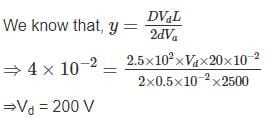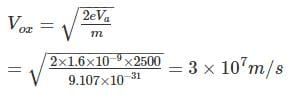Test: Electrical & Electronic Measurements- 7 - Question 6

In an analog single channel cathode ray oscilloscope (CRO), the x and y sensitivities are set as 1ms/div. and 1V/div. respectively. The y-inputs is connected to a voltage signal 4 cos (200 πt – 45°) V. The trigger source is internal, level chosen is zero and the slope is positive. The display seen on the CRO screen is

Detailed Solution for Test: Electrical & Electronic Measurements- 7 - Question 6

Input = 4 cos (200 πt – 45°)
Peak voltage = 4 V
Y sensitivity = 1V/div
No of divisions on y – axis = 4/1 = 4
ω = 200π
2π f = 200π
⇒ T = 1/100 = 10 ms
X sensitivity = 1ms/div
No. of x – divisions = 10/1 = 10 for one full cycle
The trigger is set to zero
Hence waveform starts from zero.

Test: Electrical & Electronic Measurements- 7 - Question 7

The input impedance of a CRO is equivalent to a 1 MΩ resistance in parallel with a 45 pF capacitance. It is used with a compensated 10 to 1 attenuation probe. The effective input capacitance at the probe tip is

Detailed Solution for Test: Electrical & Electronic Measurements- 7 - Question 7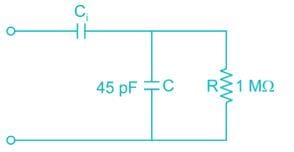Given that 10 to 1 attenuation probe.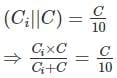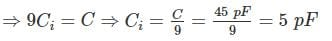Effective input capacitance = (45 || 5)
= 4.5 pF

*Answer can only contain numeric values
Test: Electrical & Electronic Measurements- 7 - Question 8

A Lissajous pattern on the oscilloscope is stationary having 8 vertical maximum values and 6 horizontal maximum values. The frequency of vertical input of the frequency of horizontal input if the frequency of horizontal input is 1800 kHz is – (in kHz)

Detailed Solution for Test: Electrical & Electronic Measurements- 7 - Question 8

Given that,
Horizontal frequency (fH) = 1800 kHz
Number of vertical tangencies (Nv) = 8
Number of horizontal tangencies (NH) = 6
We know that,
fHNH = fvNv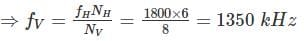*Answer can only contain numeric values
Test: Electrical & Electronic Measurements- 7 - Question 9

The below given ellipse is displayed on the screen when a CRO is operated in x-y display mode. The phase difference between both input signals is – (in degree)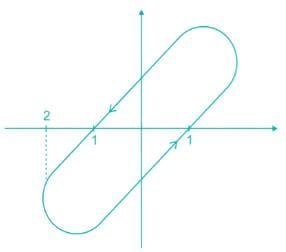Detailed Solution for Test: Electrical & Electronic Measurements- 7 - Question 9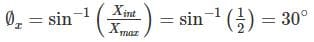f = 360°  - fx = 330°
[∵ since rotation is anticlockwise]

*Answer can only contain numeric values
Test: Electrical & Electronic Measurements- 7 - Question 10

A CRO has an anode voltage 3000 V and parallel deflection plate 3 cm long and 7 mm apart. The screen is 35 cm from the centre of the plates. Find the input voltage required to deflect the beam through 4 cm. The input voltage is applied to the deflecting plates through amplifiers having an overall gain of 100.

Detailed Solution for Test: Electrical & Electronic Measurements- 7 - Question 10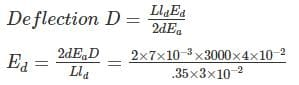= 160 V
∴ Input voltage required = 160/100 = 1.6 V

## GATE Electrical Engineering (EE) 2023 Mock Test Series

22 docs|284 tests
Information about Test: Electrical & Electronic Measurements- 7 Page
In this test you can find the Exam questions for Test: Electrical & Electronic Measurements- 7 solved & explained in the simplest way possible. Besides giving Questions and answers for Test: Electrical & Electronic Measurements- 7, EduRev gives you an ample number of Online tests for practice

## GATE Electrical Engineering (EE) 2023 Mock Test Series

22 docs|284 tests(Scan QR code)